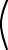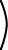det``` 1 3 -1 2 ```= 1*2 - 3*(-1) = 2+3 = 5

# Determinant of a Singular Matrix

The determinant of a 2×2 matrix is computed as follows:

 det``` a b c d ```= a*d - b*c

Computing the determinant of larger matrices is more complicated, and rarely done. The determinant is mostly used in discussing matrices, not in computing with them. The following property is often useful:

The determinant of a singular matrix is zero.

### QUESTION 14:

Compute the following determinant (just plug into the above formula):

 det``` 1 2 1 2 ```